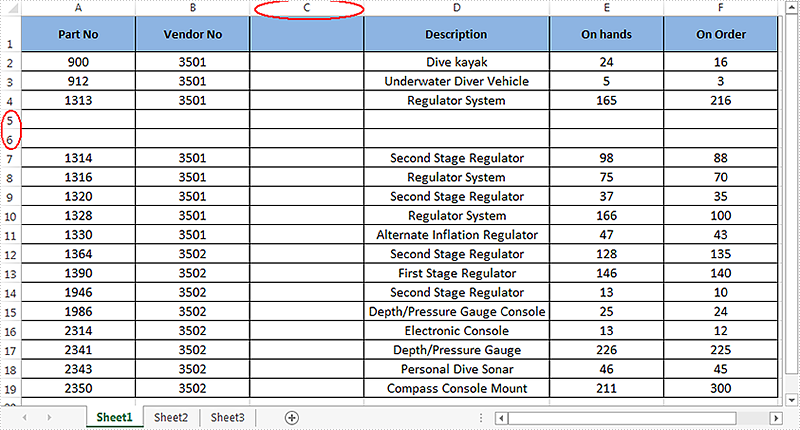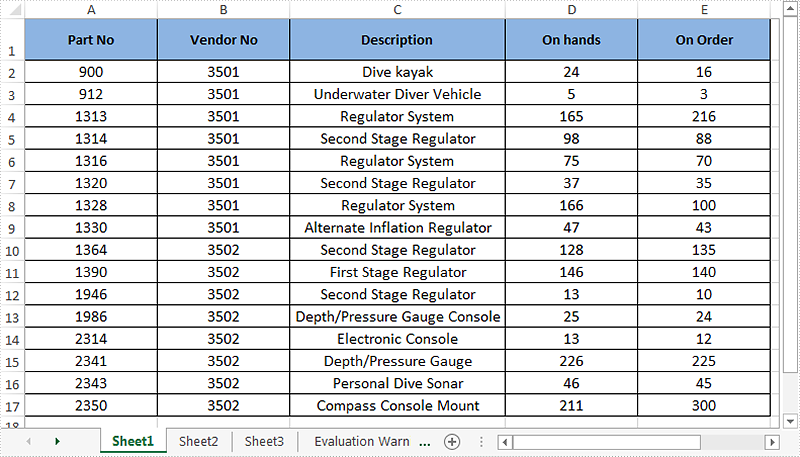# Delete Blank Rows and Columns in Excel in C#

This article demonstrates how to delete blank rows and columns in an Excel worksheet using Spire.XLS and C#.

Example Excel file:Detail steps:

Step 1: Instantiate a Workbook object and load the Excel file.

```Workbook workbook = new Workbook();
```

Step 2: Get the first worksheet.

```Worksheet sheet = workbook.Worksheets;
```

Step 3: Delete blank rows from the worksheet.

```for (int i = sheet.Rows.Count() - 1; i >= 0; i--)
{
if (sheet.Rows[i].IsBlank)
{
sheet.DeleteRow(i+1);
}
}
```

Step 4: Delete blank columns from the worksheet.

```for (int j = sheet.Columns.Count() - 1; j >= 0; j--)
{
if (sheet.Columns[j].IsBlank)
{
sheet.DeleteColumn(j+1);
}
}
```

Step 5: Save the file.

```workbook.SaveToFile("DeleteBlankRowsAndColumns.xlsx", ExcelVersion.Version2013);
```

Output:Full code:

```using System;
using System.Collections.Generic;
using System.Linq;
using System.Text;
using Spire.Xls;

namespace Delete_Blank_Rows_and_Columns_in_Excel
{
class Program
{
static void Main(string[] args)
{
//Instantiate a Workbook object
Workbook workbook = new Workbook();

//Get the first worksheet
Worksheet sheet = workbook.Worksheets;

//Delete blanks rows
for (int i = sheet.Rows.Count() - 1; i >= 0; i--)
{
if (sheet.Rows[i].IsBlank)
{
sheet.DeleteRow(i+1); //Index parameter in DeleteRow method starts from 1
}
}

//Delete blank columns
for (int j = sheet.Columns.Count() - 1; j >= 0; j--)
{
if (sheet.Columns[j].IsBlank)
{
sheet.DeleteColumn(j+1); //Index parameter in DeleteColumn method starts from 1
}
}

//Save the file
workbook.SaveToFile("DeleteBlankRowsAndColumns.xlsx", ExcelVersion.Version2013);
}
}
}
```